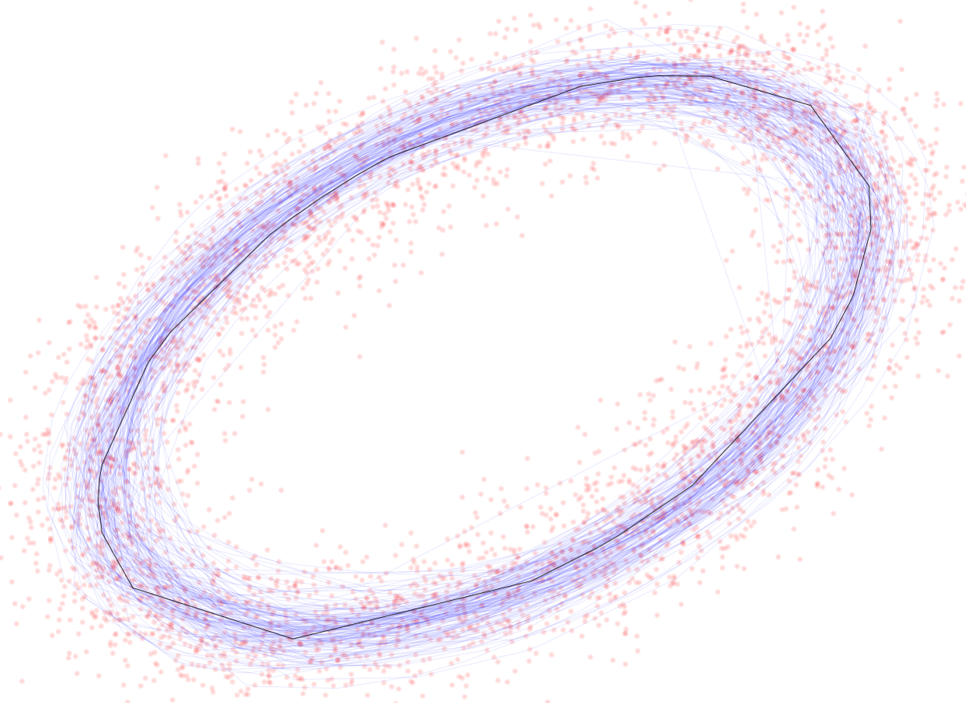# Machine Learning Cloud Regression: The Swiss Army Knife of Optimization

Entitled “Machine Learning Cloud Regression: The Swiss Army Knife of Optimization”, the full version in PDF format is accessible in the “Free Books and Articles” section, here. Also discussed in details with Python code in chapter 1 in my book “Intuitive Machine Learning and Explainable AI”, available here.

Many machine learning and statistical techniques exist as seemingly unrelated, disparate algorithms designed and used by practitioners from various fields, under various names. Why learn 50 types of regressions when you can solve your problems with one simple generic version that covers all of them and more?

The purpose of this article is to unify these techniques under a same umbrella. The data set is viewed as a cloud of points, and the distinction between response and features is blurred. Yet I designed my method to make it backward-compatible with various existing procedures. Using the same method, I cover linear and logistic regression, curve fitting, unsupervised clustering and fitting non-periodic time series, in less than 10 pages plus Python code, case studies and illustrations.

The fairly abstract approach leads to simplified procedures and nice generalizations. For instance, I discuss a generalized logistic regression with the logistic function replaced by any unspecified CDF and solved using empirical distributions. My new unsupervised clustering technique — with an exact solution — identifies the cluster centers prior to classifying the points. I compute prediction intervals even when the data has no response, in particular in curve fitting problems or for the shape of meteorites. Predictions for non periodic time series such as ocean tides are done with the same method. I also show how to adapt the method to unusual situations, such as fitting a line (not a plane) or two planes in three dimensions.

There is no statistical theory and probability distributions involved, except in the design of synthetic data to test the method. Confidence regions and estimates are based on parametric bootstrap. I provide a quick illustration for statisticians (used to a different framework) in the section “Example for Statisticians”.

## Abstract

This article is not about regression performed in the cloud. It is about considering your data set as a cloud of points or observations, where the concepts of dependent and independent variables (the response and the features) are blurred. It is a very general type of regression, offering backward-compatibility with existing methods. Treating a variable as the response amounts to setting a constraint on the multivariate parameter, and results in an optimization algorithm with Lagrange multipliers. The originality comes from unifying and bringing under a same umbrella, a number of disparate methods each solving a part of the general problem and originating from various fields. I also propose a novel approach to logistic regression, and a generalized R-squared adapted to shape fitting, model fitting, feature selection and dimensionality reduction. In one example, I show how the technique can perform unsupervised clustering, with confidence regions for the cluster centers obtained via parametric bootstrap.

Besides ellipse fitting and its importance in computer vision, an interesting application is non-periodic sum of periodic time series. While rarely discussed in machine learning circles, such models explain many phenomena, for instance ocean tides. It is particular useful in time-continuous situations where the error is not a white noise, but instead smooth and continuous everywhere. For instance, granular temperature forecast. Another curious application is modeling meteorite shapes. Finally, my methodology is model free and data driven, with a focus on numerical stability. Prediction intervals and confidence regions are obtained via bootstrapping. I provide Python code and synthetic data generators for replication purposes.Confidence region for the shape of a meteorite based on a 30 pixels image (the training set)

1 Introduction: circle fitting
. . . Previous versions of my method

2 Methodology, implementation details and caveats
. . . Solution, R-squared and backward compatibility
. . . Upgrades to the model

3 Case studies
. . . Logistic regression, two ways
. . . Ellipsoid and hyperplane fitting
. . . . . . Curve fitting: 250 examples in one video
. . . . . . Confidence region for the fitted ellipse
. . . . . . Python code
. . . Non-periodic sum of periodic time series
. . . . . . Numerical instability and how to fix it
. . . . . . Python code
. . . Fitting a line in 3D, unsupervised clustering, and other generalizations
. . . . . . Example: confidence region for the cluster centers
. . . . . . Exact solution and caveats
. . . . . . Comparison with K-means clustering

## Example for Statisticians

I provide here a comparison with standard regression on the most trivial example, for statisticians. In statistics, fitting a line is estimating a, b in y = ax + b. In my approach, it is finding a, b, c minimizing the sum of squared errors in ax +by + c = 0. There is no dependent variable. But you fall back on standard regression if you set b = -1.

You need a constraint, and a2 + b2 + c2 = 1 leads to a more elegant approach, than b = -1. The constraint results in a Lagrange multiplier in the least squares optimization. Confidence regions for (a, b, c) are obtained via bootstrap. There is no likelihood function involved. Prediction intervals are for the error between the true ax + by + c supposed to be zero by design, and the estimated one using the estimated (a, b, c), at a specific (x, y). I use the notation θ for the parameter (a, b, c).